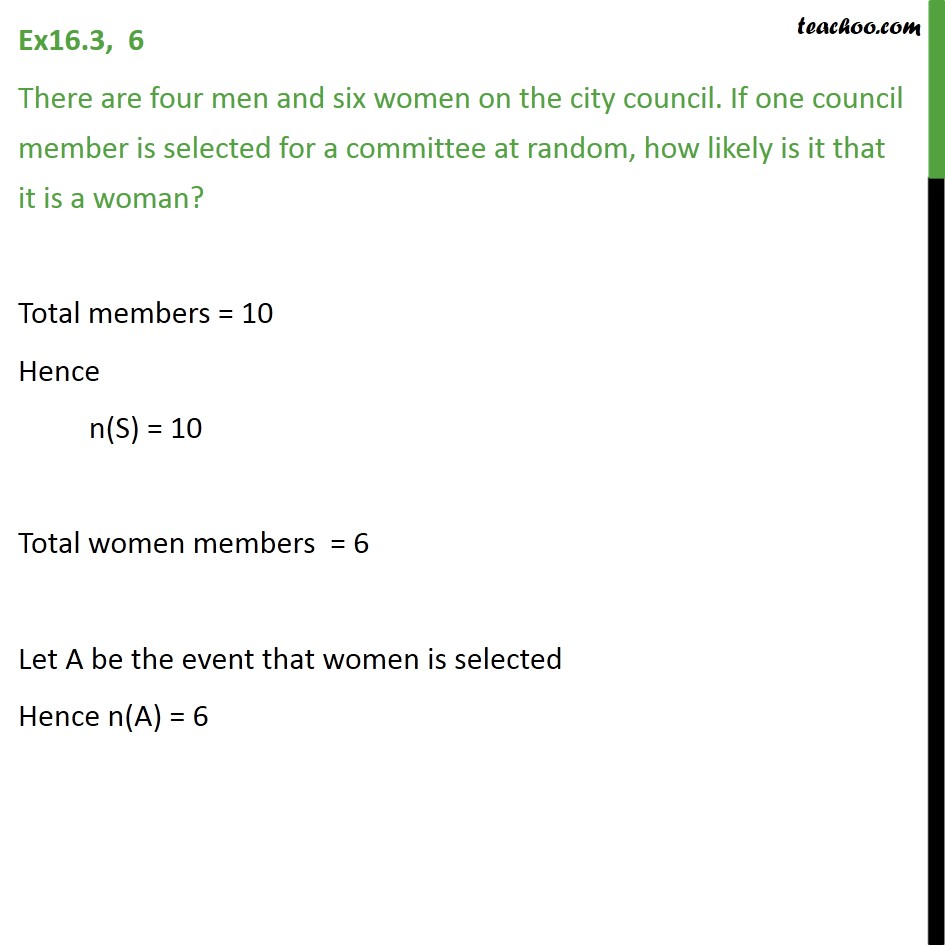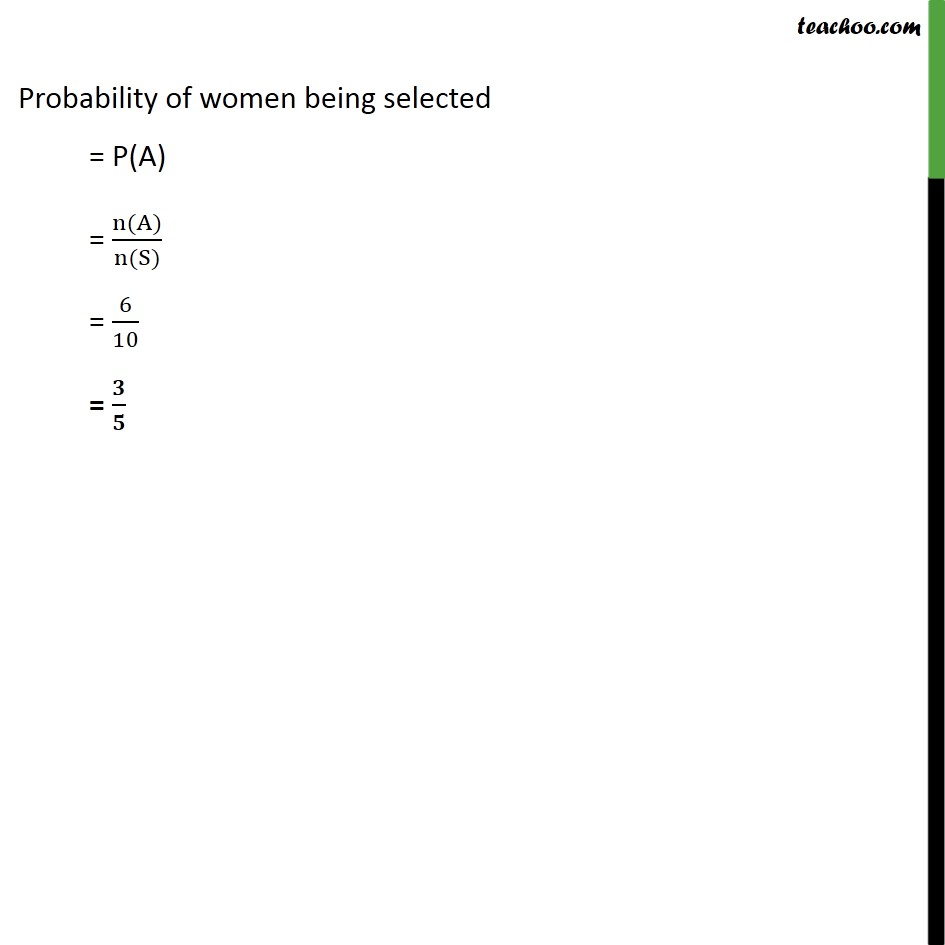1. Chapter 16 Class 11 Probability (Term 2)
2. Serial order wise
3. Ex 16.3

Transcript

Ex 16.3, 6 There are four men and six women on the city council. If one council member is selected for a committee at random, how likely is it that it is a woman? Total members = 10 Hence n(S) = 10 Total women members = 6 Let A be the event that women is selected Hence n(A) = 6 Probability of women being selected = P(A) = n(A)﷮n(S)﷯ = 6﷮10﷯ = 𝟑﷮𝟓﷯

Ex 16.3

Chapter 16 Class 11 Probability (Term 2)
Serial order wise